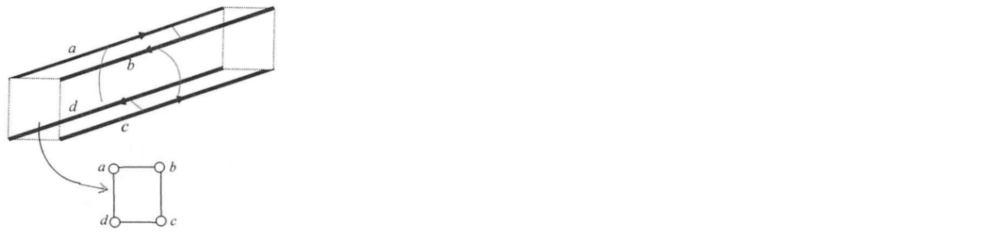# Problem: Four parallel wires, a, b, c, d, are placed at the corners of a square glass beams as shown. (The insert shows the end view from the left). Wires a and c carry parallel currents to the right and wires b and d to the left. Study all possible interactions between the currents (six interactions altogether) and tell: How many attractive interactions you find? _____ How many repulsive ones? _____

###### FREE Expert Solution
85% (220 ratings)
###### Problem Details

Four parallel wires, a, b, c, d, are placed at the corners of a square glass beams as shown. (The insert shows the end view from the left). Wires a and c carry parallel currents to the right and wires b and d to the left. Study all possible interactions between the currents (six interactions altogether) and tell:

How many attractive interactions you find? _____

How many repulsive ones? _____Frequently Asked Questions

What scientific concept do you need to know in order to solve this problem?

Our tutors have indicated that to solve this problem you will need to apply the Magnetic Force Between Parallel Currents concept. You can view video lessons to learn Magnetic Force Between Parallel Currents. Or if you need more Magnetic Force Between Parallel Currents practice, you can also practice Magnetic Force Between Parallel Currents practice problems.

How long does this problem take to solve?

Our expert Physics tutor, Juan took 2 minutes and 52 seconds to solve this problem. You can follow their steps in the video explanation above.